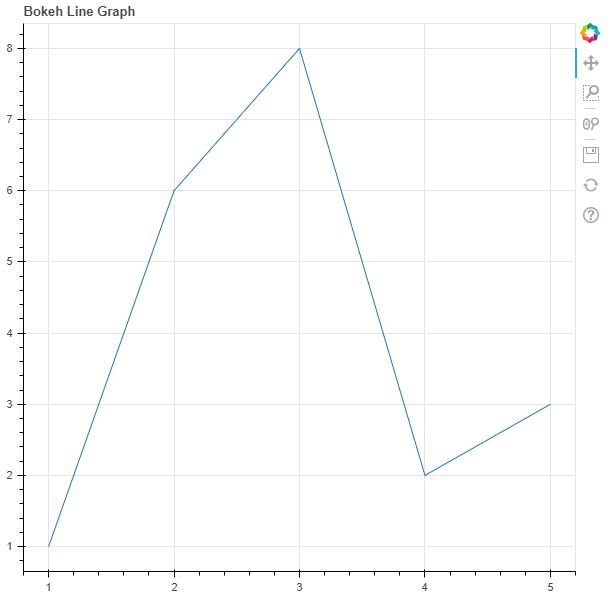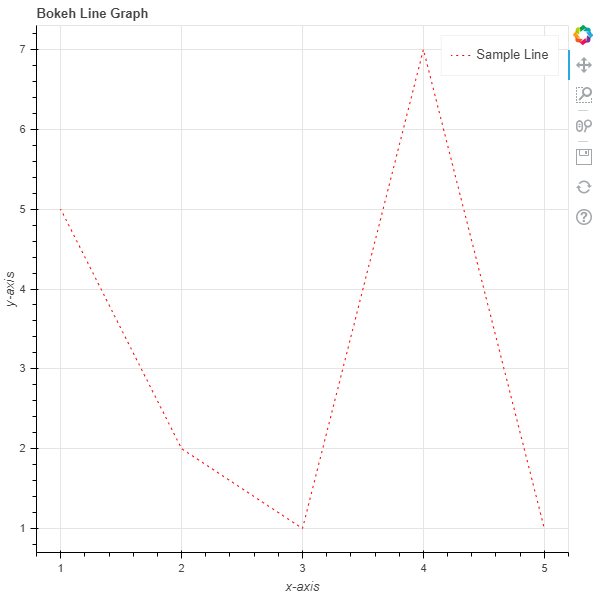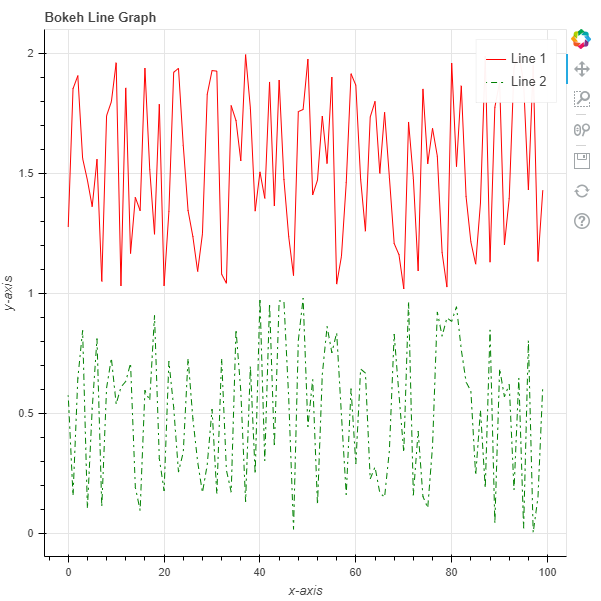GeeksforGeeks App
Open AppBrowser
Continue

# Python Bokeh – Plotting a Line Graph

Bokeh is a Python interactive data visualization. It renders its plots using HTML and JavaScript. It targets modern web browsers for presentation providing elegant, concise construction of novel graphics with high-performance interactivity.

Bokeh can be used to plot a line graph. Plotting a line graph can be done using the `line()` method of the `plotting` module.

## plotting.figure.line()

Syntax : line(parameters)

Parameters :

• x : x-coordinates of the points to be plotted
• y : y-coordinates of the points to be plotted
• line_alpha : percentage value of line alpha, default is 1
• line_cap : value of line cap for the line, default is butt
• line_color : color of the line, default is black
• line_dash : value of line dash such as :
• solid
• dashed
• dotted
• dotdash
• dashdot

default is solid

• line_dash_offset : value of line dash offset, default is 0
• line_join : value of line join, default in bevel
• line_width : value of the width of the line, default is 1
• name : user-supplied name for the model
• tags : user-supplied values for this model

Other Parameters :

• alpha : sets all alpha keyword arguments at once
• color : sets all color keyword arguments at once
• legend_field : name of a column in the data source that should be used
• legend_group : name of a column in the data source that should be used
• legend_label : labels the legend entry
• muted : determines whether the glyph should be rendered as muted or not, default is False
• name : optional user-supplied name to attach to the renderer
• source : user-supplied data source
• view : view for filtering the data source
• visible : determines whether the glyph should be rendered or not, default is True
• x_range_name : name of an extra range to use for mapping x-coordinates
• y_range_name : name of an extra range to use for mapping y-coordinates
• level : specifies the render level order for this glyph

Returns : an object of class `GlyphRenderer`

Example 1 :In this example we will be using the default values for plotting the graph.

 `# importing the modules``from` `bokeh.plotting ``import` `figure, output_file, show``  ` `# file to save the model``output_file(``"gfg.html"``)``  ` `# instantiating the figure object``graph ``=` `figure(title ``=` `"Bokeh Line Graph"``)`` ` `# the points to be plotted``x ``=` `[``1``, ``2``, ``3``, ``4``, ``5``]``y ``=` `[``1``, ``6``, ``8``, ``2``, ``3``]``  ` `# plotting the line graph``graph.line(x, y)``  ` `# displaying the model``show(graph)`

Output :Example 2 :In this example we will be plotting a line graph with dotted lines alongside other parameters.

 `# importing the modules``from` `bokeh.plotting ``import` `figure, output_file, show``  ` `# file to save the model``output_file(``"gfg.html"``)``  ` `# instantiating the figure object``graph ``=` `figure(title ``=` `"Bokeh Line Graph"``)`` ` `# name of the x-axis``graph.xaxis.axis_label ``=` `"x-axis"`` ` `# name of the y-axis``graph.yaxis.axis_label ``=` `"y-axis"`` ` `# the points to be plotted``x ``=` `[``1``, ``2``, ``3``, ``4``, ``5``]``y ``=` `[``5``, ``2``, ``1``, ``7``, ``1``]`` ` `# color of the line``line_color ``=` `"red"`` ` `# type of line``line_dash ``=` `"dotted"`` ` `# offset of line dash``line_dash_offset ``=` `1`` ` `# name of the legend``legend_label ``=` `"Sample Line"`` ` `# plotting the line graph for AAPL``graph.line(x, y,``           ``line_color ``=` `line_color,``           ``line_dash ``=` `line_dash,``           ``line_dash_offset ``=` `line_dash_offset,``           ``legend_label ``=` `legend_label)``  ` `# displaying the model``show(graph)`

Output :Example 3 :Now we will see how to plot multiple lines in the same graph. We will generate the points using the `random()` function.

 `# importing the modules``from` `bokeh.plotting ``import` `figure, output_file, show``import` `random`` ` `# file to save the model``output_file(``"gfg.html"``)``  ` `# instantiating the figure object``graph ``=` `figure(title ``=` `"Bokeh Line Graph"``)`` ` `# name of the x-axis``graph.xaxis.axis_label ``=` `"x-axis"`` ` `# name of the y-axis``graph.yaxis.axis_label ``=` `"y-axis"`` ` `# plotting line 1``# generating the points to be plotted``x ``=` `[]``y ``=` `[]``for` `i ``in` `range``(``100``):``    ``x.append(i)``for` `i ``in` `range``(``100``):``    ``y.append(``1` `+` `random.random())`` ` `# parameters of line 1``line_color ``=` `"red"``line_dash ``=` `"solid"``legend_label ``=` `"Line 1"`` ` `# plotting the line``graph.line(x, y,``           ``line_color ``=` `line_color,``           ``line_dash ``=` `line_dash,``           ``legend_label ``=` `legend_label)`` ` `# plotting line 2``# generating the points to be plotted``x ``=` `[]``y ``=` `[]``for` `i ``in` `range``(``100``):``    ``x.append(i)``for` `i ``in` `range``(``100``):``    ``y.append(random.random())`` ` `# parameters of line 2``line_color ``=` `"green"``line_dash ``=` `"dotdash"``line_dash_offset ``=` `1``legend_label ``=` `"Line 2"`` ` `# plotting the line``graph.line(x, y,``           ``line_color ``=` `line_color,``           ``line_dash ``=` `line_dash,``           ``line_dash_offset ``=` `line_dash_offset,``           ``legend_label ``=` `legend_label)``  ` `# displaying the model``show(graph)`

Output :My Personal Notes arrow_drop_up